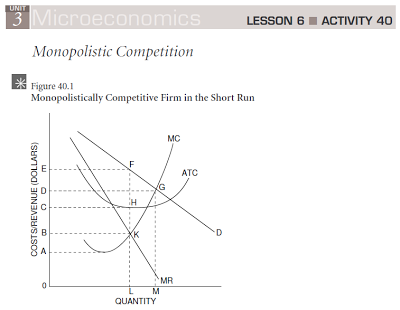APE 40-Monopolistic Competition
First Name *
Last Name *
School email address (type in carefully) *
Class Period *
1: Use the graph to answer questions A-H
(A) At what level of output will this firm operate? *
1 point(B) What is marginal revenue at this level of output? *
1 point(C) What price will this firm charge for its product? *
1 point(D) The area of which rectangle is equal to total revenue? *
1 point(E) What is the firm’s average total cost? *
1 point(F) The area of which rectangle is equal to the firm’s total cost? *
0 points(G) Is the firm in the short run or long run? *
1 pointHow do you know if the firm is in the short run or long run? *
0 points
(H) The area of which rectangle is equal to profits or losses? *
0 points2. Would the demand curve for a monopolistic competitor be more or less elastic than the demand curve for a monopolist? Justify your answer. *
0 points
3. What are the characteristics of a monopolistically competitive market? In what sense is there competition and in what sense is there monopoly in this type of market structure? *
0 points
4. What are three examples of monopolistically competitive markets? *
0 points